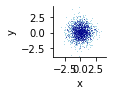# Matplotlib + datashader plot sizes?

The dimensions of the matplotlib plot are determined by the range of the values plotted. How can I specify them separately? With the bokeh backend this would be done with `width` or `height`. I had assumed the correct value would be `fig_size`, but these appears to only take scalar values.

Here’s an example of what’s going wrong:

``````import holoviews as hv
import numpy as np
import pandas as pd

hv.extension("matplotlib")

df = pd.DataFrame({"x": np.random.randn(100000), "y": np.random.randn(100000)}, index=range(100000))

# This looks okay:
``````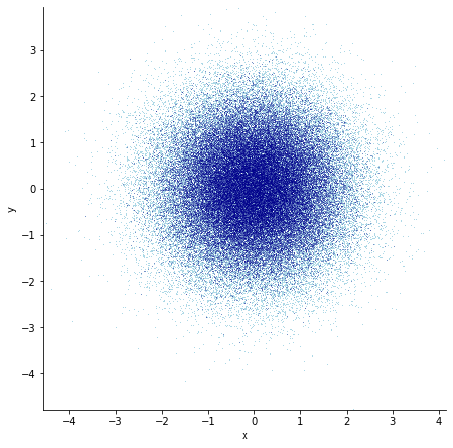``````# This does not:
df["x2"] = df["x"] * 10

``````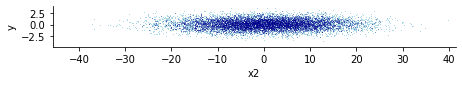Found my solution! There is an `aspect` option. While the `fig_inches` option also sounds like it should work, as of `holoviews v1.12.7`, it does not.

Here’s an example of usage:

``````display(datashade(hv.Points(df, ["x2", "y"])).opts(fig_size=200, aspect=1))
``````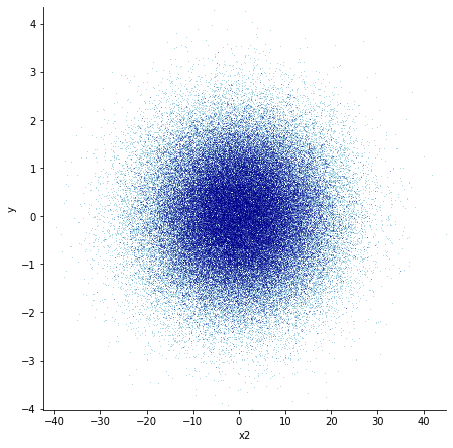Glad you found something that works! That said, I’ll try to see if I can reproduce ` fig_inches` not working…afaik it should…

Here’s an example of the `fig_inches` behaviour:

``````import holoviews as hv
import numpy as np
import pandas as pd

hv.extension("matplotlib")

df = pd.DataFrame({"x": np.random.randn(100000), "y": np.random.randn(100000)}, index=range(100000))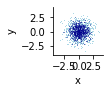``````display(datashade(hv.Points(df, ["x", "y"])).opts(fig_inches=(3, 1)))